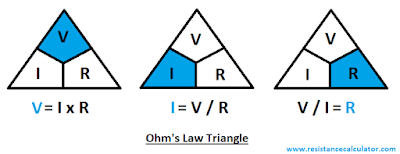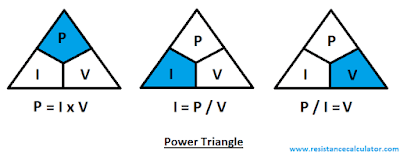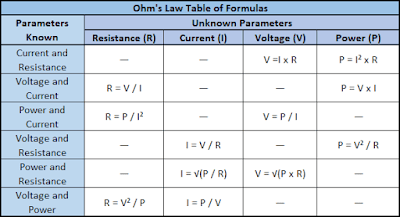## Ohm's law Calculator

The Ohm's law Calculator along with calculations are presented here which will ease your design and circuit calculations.

For calculations of Resistor for LED please use, Resistor for LED Calculator.

## Ohm’s law Calculator

Enter any two known values and press "Calculate" to get answer and "Reset" to clear.
Volts
Amps
Ohms
Watts

Many times many of us heard about Ohm’s law. All electrical and electronics engineer, technicians, hobbyist, etc. normally or frequently deals with ohm’s law, that’s why it’s very important to understand ohm’s law.

Here in this article Ohm’s law definition, Ohm’s law calculator and calculations are presented and discussed in detail.

Ohm’s law calculations will be easy with the help of this Ohm’s law calculator. You can compare manually calculated values using ohm’s law formula with the results of this calculator.

### Ohm’s law Calculations and Calculator Excel Sheet

An excel sheet for Ohm's law calculation is provided which you can get by clicking on below button. This excel sheet you can use in your laptop, computer, tablet or mobile. Please click below button to download it.
Now let discuss Ohm’s law definition and its calculation in detail;

### What is Ohm’s law

We can define or we can say the definition of Ohm’s law is, “the current across a conductor between two points is directly proportional to the voltage across the two points”.

Resistance is introduced as constant of proportionality. Resistance in given equation is constant and not dependent of the current.

Ohm law is invented by the German physicist Georg Ohm. As per Georg Ohm; for a constant or continual temperature, the current flowing across or over a firm or fixed linear resistance is directly proportional (corresponding) to the voltage applied across (over) it, and inversely proportional (corresponding) to the resistance.

In other words, or simple words, we can also say that; the relationship between Voltage, Current and Resistance is called as Ohm’s law.

Ohm's law is an empirical relation i.e. experimental relation which exactly defines the conductivity of the several or many of electrically conductive materials over different level or stages of the current.

Non-ohmic material or conductors do not follow Ohm's law.

### Ohm’s law equation

The equation in terms of math for the Ohm’s law can be given as; Resistance = Voltage / Current.

Where,
Current (I) = current across or through the conductor, having the units of amperes.
Voltage (V) = voltage measured across or through the conductor, having the units of volts.
Resistance (R) = resistance of the conductor, having the units of ohms.

Ohm’s law formula is;

For voltage calculation, V
V = I x R

For current calculation, I
I = V / R

For resistance calculation, R
R = V / I

### Ohms law triangle

Ohm’s law triangle helps us to remember the ohm’s law formula's by the help of picture.

In this triangle the voltage, current and resistance conquers or occupies different spaces (places) and all are fixed in these spaces i.e. no variation or deviation of spaces is allowed.

In this ohm’s law triangle initial position starts with Voltage which is at top. Below position is occupied by Current and Resistance.

According to the Ohm’s law the voltage, current and resistance positions are fixed in the triangle i.e. the positions are according to the Ohm’s law.

Below triangles explains the Ohm’s law in easy way;Ohms Law Triangle

### Electric Power and Ohm’s law

The rate per unit time, an electric circuit transfers electrical energy can be defined as Electric Power. The International System of Units i.e. SI unit of the power is Watt one joule per second.

Letter “P” is used to represent it.

The Power can be given as; “P = V x I” in terms of voltage and current.

Electric Power formula using ohm’s law

Electric power formula, we can write as per ohm’s law;

P = V² ÷ R
P = I² x R

### Power Triangle

Below picture will help us to remember the formula for power, this picture is called as power triangle.

In this triangle the power, current and voltage conquers or occupies different spaces (places) and all are fixed in these spaces i.e. no variation or deviation of spaces is allowed.

In this power triangle initial position starts with Power, which is at top. Below position is occupied by Current and Voltage.

According to the Ohm’s law the voltage, current and resistance positions are fixed in the triangle i.e. the positions are according to the Ohm’s law.

Below triangles explains the electrical power formula's in easy way;Power Triangle

### Ohm’s law wheel

Ohm’s law formula and electric power formula now we know very well.

By these formulas, we can create ohms’ law wheel. Twelve different formulas are mentioned in ohms’ law wheel according to Voltage, Current, Power, and Resistance.

Also, Ohm's law Table of Formulas we can create on the basis of the same;Ohms Law Table of Formulas

### Conclusion

Ohm's law is a significant rule to answer or explain the current, voltage, resistance and power issues.

The calculator and calculations presented here will help to calculate current, voltage, resistance, power. These calculations surely will help you to understand the concept of Ohm's law. Here the calculation process will be easy with the help of presented calculator.